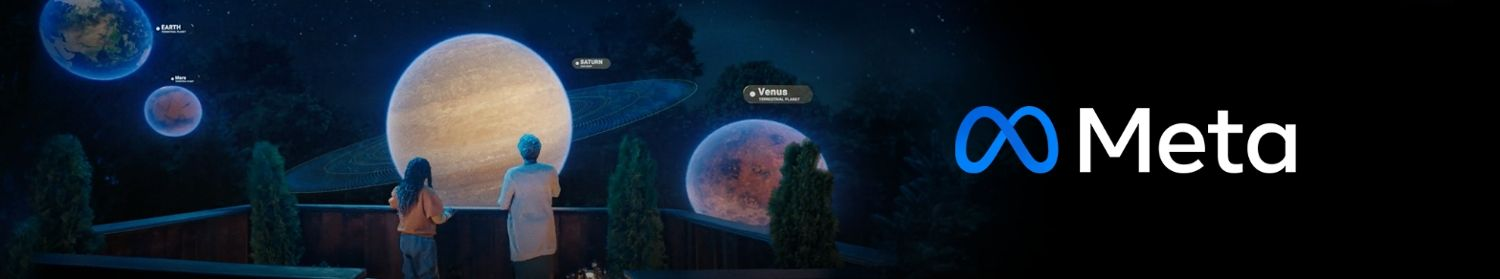Meta

## Interview Question

Front End Engineer Interview

-

# Implement a simple store class with set(Node, value), get(Node) and has(Node) methods, which store a given Nodes with corresponding values.

3

Since node is object we can store value directly on the node itself. But it become complex and may mess with original object. Luckily we have Symbol come to rescue: class DOMStore { constructor () { this.DOMStoreSymbol = Symbol('DOMStore'); } has (node) { return node[this.DOMStoreSymbol] !== undefined; } set (node, value) { node[this.DOMStoreSymbol] = value; } get (node, defaultValue) { return node[this.DOMStoreSymbol] || defaultValue; } } const e1 = document.createElement('A'); const e2 = document.createElement('P'); const e3 = document.createElement('DIV'); const e4 = document.createElement('TABLE'); const e5 = document.createElement('A'); const map1 = new DOMStore(); const map2 = new DOMStore(); console.assert(map1.has(e1) === false, 'map1 should be empty'); map1.set(e1, 'E1 in map1'); console.assert(map1.has(e1) === true, 'map1 must have e1'); map2.has(e1); console.assert(map2.has(e1) === false, 'map2 should be empty'); map1.set(e2, 1234); map1.set(e3, "String"); map1.set(e4, [1,2,3,4]); console.assert(map1.get(e5, null) === null); console.assert(map1.get(e5) === undefined); console.assert(map1.get(e5, 5) === 5); map1.set(e5, {1: 2, 3: 4}); console.assert(map1.get(e1) === 'E1 in map1'); console.assert(typeof(map1.get(e5)) === 'object');

Hacky O(1) solution :D just for fun. on

5

Are you able to elaborate on this question? Can each node have multiple values or just 1? The way I interpret what you have written, it just sounds like they are asking for an ES6 Map? ES6 Maps can have objects as keys, so you can use the Node as the key. If you had to code it from scratch without the use of an ES6 Map, something like: class CachedNode { constructor(node, value) { this._node = node; this._value = value; } getNode() { return this._node; } getValue() { return this._value; } setValue(value) { this._value = value; return this; } } class SimpleStore { constructor() { this._container = []; } set(node, value) { let cachedNode; if (this.has(node)) { cachedNode = this.get(node); cachedNode.setValue(value); } else { cachedNode = new CachedNode(node, value); this._container.push(cachedNode); } return this; } // you might want to change this method so it returns the Node's value not the CachedNode. // If you did, that would mean adding another method to get the CachedNode. Easy enough. get(node) { return this._container.find((cachedNode) => { return cachedNode.getNode() === node; }); } has(node) { return !!this.get(node); } } If it needed to store multiple values against a node, then just change the single value for a Set or Array.

Anonymous on

0

The tricky part of the question was on how to store a DOM Node which is an object as an old javascript object key. I can't recall was it about 1 to 1 relationship or 1 to many, but it is really doesn't matter because the later gives just a small overhead. I suppose your solution is pretty what they were expected from the task, starting from explaining ES6 Map and ending up with an old javascript solution.

Valerii on

0

``` let Store = Map; ``` The problem listed the exact behavior and method signature of the Map class. Must be some trick question.

Anonymous on

0

They want you to implement ES6 Map. One of the answers given above comes close, but it doesn't really need to compare cachedNode.getNode() === node. The trick is that objects are passed by reference, and if you just created an array, and added the node as an item there, you could use arr.include(node) for `has` method of the store. Also, you can have 2 arrays, storeArray = [node1, node2, node3, node4]; valueArray = [1,2,3,4]; So `store.has(node3)`, you would do this._storeArray.includes(node3); // true! No comparison needed due to pass by reference of JS. and for `store.get(node3)` return this._valueArray(this._storeArray.indexOf(node3));

Om Shankar on

0

class Store{ constructor(){ this._map = new WeakMap(); } set(node, value){ this._map.set(node,value); } get(node){ return this._map.get(node); } has(node){ return this._map.has(node); } } const store = new Store(); const node1 = document.createElement("div") const node2 = document.createElement("div") const node3 = document.createElement("div") const node4 = document.createElement("div") store.set(node1,1); store.set(node2,2); store.set(node3,3); store.get(node1) // 1 store.get(node2) // 2 store.get(node3) // 3 store.has(node1) // true store.has(node4) // false

YoYo on

0

class NodeStore { constructor() { this.store = {} } /** * @param {Node} node * @param {any} value */ set(node, value) { const nodeKey = Symbol(node) node.symbol = nodeKey this.store[nodeKey] = value } /** * @param {Node} node * @return {any} */ get(node) { const nodeKey = node.symbol return this.store[nodeKey] } /** * @param {Node} node * @return {Boolean} */ has(node) { return this.store[node.symbol] !== undefined } }

Anonymous on